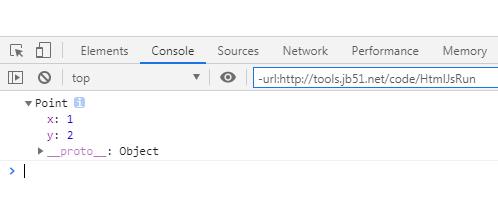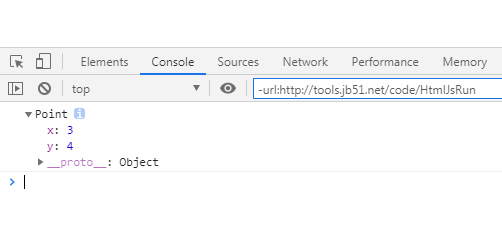# js定义类的方法示例【ES5与ES6】

``````
<script>
function Point(x,y){
this.x=x;
this.y=y;
}
Point.prototype.toString=function(){
return '('+this.x+', '+this.y+')';
}
var point=new Point(1,2);
console.log(point);
</script>

``````es6里简化了类的定义方法：

``````
<script>
class Point{
constructor(x,y){
this.x=x;
this.y=y;
}
toString(){
return '('+this.x+', '+this.y+')';
}
}
let point=new Point(3,4);
console.log(point);
</script>

``````